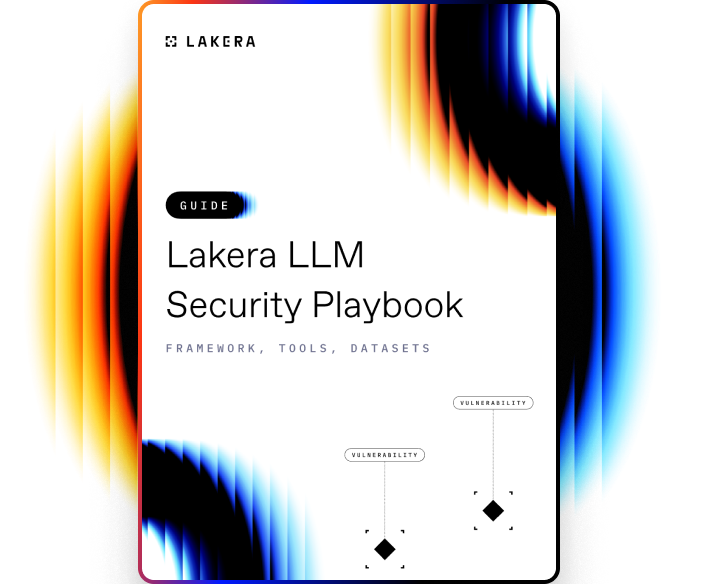VOCABULARY

# Learning Rate

The learning rate in Machine Learning (ML) is a configuration parameter that determines how much an algorithm changes its model in response to the estimated error each time the model weights are updated. In essence, it defines the speed at which a machine learning model 'learns'.

The learning rate in Machine Learning (ML) is a configuration parameter that determines how much an algorithm changes its model in response to the estimated error each time the model weights are updated. In essence, it defines the speed at which a machine learning model 'learns'. It is often represented by the Greek letter η and is a key parameter in the configuration of many machine learning algorithms.

## How Learning Rate works

In gradient descent algorithms, which aim to minimize a given cost function, the learning rate controls how big the step would be on each iteration. When the learning rate is low, the model makes small updates to the weights and requires more iterations to converge to the solution, leading to a slow training process. On the other hand, when the learning rate is high, the model makes larger updates to the weights, which might lead the model to overshoot the optimal solution and may even fail to converge.

It is pivotal to note that setting the learning rate is quite critical, since a poorly chosen learning rate can result in the model learning too slowly, getting stuck in a suboptimal solution, or even diverging entirely from the optimal solution.

The process of determining the best learning rate often involves experimentation; one common technique is using a learning rate schedule or adaptive learning rates that start with a high learning rate and reduce it over time, allowing the model to make large changes in the beginning and more detailed refinements as the training progresses. This helps to balance between the speed of learning and the risk of overshooting or failing to converge to the optimal solution.Learn how to protect against the most common LLM vulnerabilities

Download this guide to delve into the most common LLM security risks and ways to mitigate them.

Related termsActivate
untouchable mode.